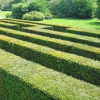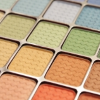#### You may also likeThis comes in two parts, with the first being less fiendish than the second. Itâ€™s great for practising both quadratics and laws of indices, and you can get a lot from making sure that you find all the solutions. For a real challenge (requiring a bit more knowledge), you could consider finding the complex solutions.### Discriminating

You're invited to decide whether statements about the number of solutions of a quadratic equation are always, sometimes or never true.This will encourage you to think about whether all quadratics can be factorised and to develop a better understanding of the effect that changing the coefficients has on the factorised form.

# Equation or Identity (2)

##### Age 16 to 18Challenge LevelThis resource is from Underground Mathematics.

Throughout, $A$, $B$ and $C$ are the angles of a triangle.

For each of the following, decide whether it is an identity (true for all triangles) or an equation (there is a triangle for which it is not true).

If it is an identity, true for all triangles, then you should prove it (using trigonometric identities that you already know).

If it is an equation, then give an example of a triangle for which it is not true.  You could also try to solve the equation (that is, find all triangles for which it is true).

1.  $\sin(A + 2B) = \sin A + 2\sin B \cos(A + B)$.

2.  $\tan(A - B) + \tan(B - C) + \tan(C - A) = 0$.

3.  $2\sin A \cos^2\left(\frac{B}{2}\right) + 2\cos^2\left(\frac{A}{2}\right)\sin B = \sin(A + B) + \sin(B + C) + \sin(C + A)$.

4.  $\sin(A+B) = \cos C$.

5.  $\cos C = -\cos(A+B)$.

6.  $4(\cos^2 A \cos^2 B + \sin^2 A \sin^2 B) - 2 \sin(2A) \sin(2B) = 3$.

7.  $\sin(2A) + \sin(2B) + \sin(2C) = 4\sin A \sin B \sin C$.

This is an Underground Mathematics resource.

Underground Mathematics is hosted by Cambridge Mathematics. The project was originally funded by a grant from the UK Department for Education to provide free web-based resources that support the teaching and learning of post-16 mathematics.

Visit the site at undergroundmathematics.org to find more resources, which also offer suggestions, solutions and teacher notes to help with their use in the classroom.